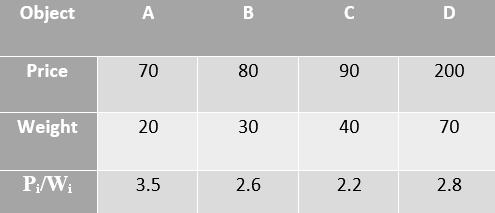# Greedy Algorithms

The greedy algorithm follows the problem-solving heuristic of making the locally optimal choice at each stage. A greedy strategy does not always produce an optimal solution. Still, a greedy heuristic may give an optimal solution.

We start by assuming a problem scenario, where we are packing things for a trek; we aim to pack items that are helpful for the hike and also to try to keep our knapsack as light as possible because hiking with lighter luggage is more fun!😛

So, the optimal solution is to carry the most valuable things which are not that heavy.

There are three approaches to this:

1. Being greedy on profit

2. Being greedy on weight

3. Being greedy on Pᵢ/Wᵢ ratio

To proceed further, let’s assume a practical scenario where we are packing things for a trek, and we have four objects {A, B, C, D} with the corresponding weights,

W={20,30,40,70}

And every object has a priority say on a scale of 200,

P={70,80,90,200}

And also maximum capacity of our knapsack is 60.

# Greedy on Profit:

In this approach we add the objects into the knapsack based on their priority irrespective of all other factors i.e. The object with highest priority goes into the knapsack first.

O ={A,B,C,D}

W={20,30,40,70}

P={70,80,90,200}

C = 60

So in our case, the object with the highest priority is ‘D,’ so we try to add D into our sack, but D exceeds the maximum capacity of our sack,

so we try to add the object with the next highest priority, that is object ‘C’ weight of object C is less than maximum capacity, so we add C,

After adding C into the sack,

Capacity = (Remaining Capacity)-(Weight of the object added)

Capacity = 60–40 = 20

Now we try to add the object with the next highest priority, that is, object ‘B’ weight of object ‘B’ exceeds the remaining capacity, so we search for the object with the next highest priority, which is the object ‘A’

We add A into the sack,

Capacity = 20–20 = 0

So, at the end contents of our knapsack are,

{C, A}

Weight of Knapsack at the end (ΣWᵢ) = 40 + 20 = 60

Profit/Priority Score (ΣPᵢ) = 90 + 70 = 160

# Greedy on Weight:

We always try to keep our knapsack as light as possible, because hiking with lighter luggage is more fun!😛

In this approach, we start filling the knapsack with the lowest weight, so the object with the lowest weight goes into the knapsack first, irrespective of all other factors.

O ={A,B,C,D}

W={20,30,40,70}

P={70,80,90,200}

C = 60

So in our case, the object with the lowest weight is ‘A,’ so we add A into our sack,

Capacity = (Remaining Capacity)-(Weight of the object added)

Capacity = 60–20 = 40

Now we try to add the object with the next lowest weight, that is, object ‘B’ weight of object B is less than maximum capacity, so we add B,

After adding B into the sack,

Capacity = (Remaining Capacity)-(Weight of the object added)

Capacity = 40–30 = 10

Since there are no more objects lesser than the capacity, we stop adding, and we end packing,

Now we have,

{A,B} in our sack

Weight of Knapsack at the end (ΣWᵢ) = 20 + 30 =50

Profit/Priority Score (ΣPᵢ) = 70 + 80 = 150

# Greedy on (Pᵢ/Wᵢ) ratio:(Pᵢ/Wᵢ) ratio

In this version, items can be broken into smaller pieces. So, we may take only a fraction x of iᵗʰ item.

0⩽xᵢ⩽10⩽xᵢ⩽1

The iᵗʰ item contributes the weight x.wᵢ to the total weight in the knapsack and profit x.pᵢ to the total profit.

Hence, the objective of this algorithm is to maximize,

∑(xᵢ.pᵢ)

subject to constraint,

∑(xᵢ.wᵢ)⩽ W

It is clear that an optimal solution must fill the knapsack exactly, otherwise we could add a fraction of one of the remaining items and increase the overall profit.

Thus, an optimal solution can be obtained by

∑(xᵢ.wᵢ)⩽ W

In this context, first we need to sort those items according to the value of Pᵢ/Wᵢ so that,

(Pᵢ+1)/(Wᵢ+1)⩽Pᵢ/Wᵢ

So now we start filling the knapsack with highest (Pᵢ/Wᵢ) ratio so the object with (Pᵢ/Wᵢ) ratio goes into the knapsack first, irrespective of all other factors.

O ={A,B,C,D}

W={20,30,40,70}

P={70,80,90,200}

C = 60

So now we start filling the knapsack with highest (Pᵢ/Wᵢ) which is ‘A’ so we add ‘A’,

Capacity = (Remaining Capacity)-(Weight of the object added)

Capacity = 60–20 = 40

Now, the object with next highest Pᵢ/Wᵢ ratio is ‘D’ , but we cant add ‘D’ because remaining capacity is less than weight of ‘D’

So, the object with next highest Pᵢ/Wᵢ ratio is ‘B’ so we add ‘B’,

After adding B into the sack,

Capacity = (Remaining Capacity)-(Weight of the object added)

Capacity = 40–30 = 10

Since, there are no more objects lesser than the capacity we stop adding and we end packing,

Now we have,

{A,B} in our sack

Weight of Knapsack at the end (ΣWᵢ) = 20 + 30 =50

Profit/Priority Score (ΣPᵢ) = 70 + 80 = 150

Major in Computer Science and Communication

## More from Sai Rohith T

Major in Computer Science and Communication